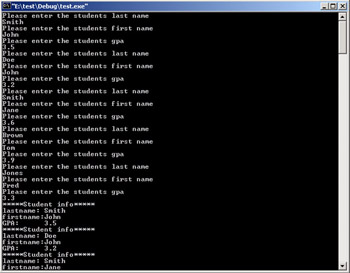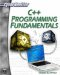# Arrays and Pointers with Classes

It is important that you realize one important fact, once you have created a class, it is really a new data type, much like the existing data types (int, float, bool, etc.). Clearly the class is much more complex than an int or float, but it is still just a data type, and anything you can do with a standard data type you can do with a class. This means that you can create arrays of classes and pointers to classes. The following example shows the use of an array of classes. This example also uses a pointer to the array of classes, to input the data into the class.

 Hint! The array of classes in this example is rather small, that makes it easier for you to input data.

### Example 10.8

Step 1: Write the following code into your favorite text editor.

`#include <iostream> using namespace std;   class student { public:  char lastname;  char firstname;  float gpa;  void displaydata(); };   void student::displaydata() {  cout << "*****Student info*****\n";  cout << "lastname: " << lastname << endl; cout << "firstname:" << firstname << endl;  cout << "GPA: " << gpa << endl; } int main() { student mystudents; student *ptr = mystudents; int i; for (i = 0;i<5;i++) {   cout << "Please enter the students last name \n";   cin>>ptr->lastname;       cout << "Please enter the students first name \n";   cin >> ptr->firstname;       cout << "Please enter the students gpa \n";   cin >> ptr->gpa;       ptr++; }// end of for loop     for(i=0;i<5;i++) {   mystudents[i].displaydata(); } return 0; }// end of main`

Step 2: Compile and execute the code. You should see something much like what is depicted in Figure 10.8.Figure 10.8: Pointers and arrays with classes.

An array of classes is not much different from an array of integers. All the rules that normally apply to arrays still apply. The same is true of pointers. When you create a class, it will occupy a certain amount of memory. When you create a pointer to a class, it points to the first byte of memory occupied by that class.

One final note to remember is the keyword: this. In most object-oriented programming languages, this refers to the current instance of a class you are in. If you used code such as

`this.funca();`C++ Programming Fundamentals (Cyberrookies)
ISBN: 1584502371
EAN: 2147483647
Year: 2005
Pages: 197
Authors: Chuck Easttom